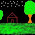### Math misconceptions

It's very good to know something about the most common misconceptions students might have.
The website CountOn.org has 22 examples of them at http://www.counton.org/resources/misconceptions/.

Here are some examples:

#2. Multiplication always increases a number... is that really so?

Well, to small kids it appears to be so - but only if you just try whole numbers. Take 10 for example. If you multiply it by 2, 3, 4, 5, etc., it does get bigger.

But all you have to do is multiply it by a fraction less than 1, or by a negative number, and 10 does not get bigger... 1/2 x 10 is 5. 1/4 x 10 is 2.5. -3 x 10 is -30.

We must remember that repeated addition is not the only meaning or definition for multiplication. That's what it is for whole numbers.

For fractions, 1/3 x 12 is better understood as 1/3 of 12.

#3. As 1 x 1 = 1, then 0.1 x 0.1 = 0.1.
This one was new to me. Quite curious. A child might think of 0.1 as some kind of "unit" like 1.

But seriously, 0.1 is one tenth. Taking tenth part of a tenth is a hundredth, or 0.01.

#5. 3 divide 1/4 is same as 3 divide 4.
This must be due to forgetting the second part of the "invert and multiply" rule. If you remember to think of division as "how many times does the divisor fit into the dividend?", then it's easy and clear: 1/4 fits into 3 exactly 12 times.

#7. What is the value that satisfies -4 + ___ = -10 ? Many possible wrong answers, such as 6, 14, or -14.

It's a missing addend problem. We already have -4, and end up with much more negatives, namely -10. So the debt increased, by -6.

#20: 2.3 x 10 = 2.30 ? 20.3 ?
Well, multiply in parts: 10 x 2 and 10 x 0.3. You get 20 and 3, total 23.

These and more are presented at Counton.org/resources/misconceptions/. They also have a one single PDF file that contains them all.

Another site listing popular (or common) math mistakes is MathMistakes.info.

Tags: ,Anonymous said…
I think the thing with misconception #2 is that most students -- even my upper-level math majors -- automatically think "integer" when they hear the word "number". Ask somebody to pick a number between 1 and 10; I guarantee you nobody will pick 1.2 or sqrt(3). Ask somebody to pick a number between 0 and 1 and chances are they'll look at you funny.I am seeing your articles on Maths recently, really good articles. I am also interested in teaching mathematics and loving Mathematics very much. I am well versed in Vedic Mathematics(of our India) , the speed calculation method in mathematics. I also request you to view my articles on mathematics, specailly jokes on mathematics: my blog is : http://khvmathematics.blogspot.com/